# griddedInterpolant

Gridded data interpolation

## Description

Use `griddedInterpolant` to perform interpolation on a 1-D, 2-D, 3-D, or N-D gridded data set. `griddedInterpolant` returns the interpolant `F` for the given dataset. You can evaluate `F` at a set of query points, such as `(xq,yq)` in 2-D, to produce interpolated values `vq = F(xq,yq)`.

Use `scatteredInterpolant` to perform interpolation with scattered data.

## Creation

### Syntax

``F = griddedInterpolant``
``F = griddedInterpolant(x,v)``
``F = griddedInterpolant(X1,X2,...,Xn,V)``
``F = griddedInterpolant(V)``
``F = griddedInterpolant(gridVecs,V)``
``F = griddedInterpolant(___,Method)``
``F = griddedInterpolant(___,Method,ExtrapolationMethod)``

### Description

````F = griddedInterpolant` creates an empty gridded data interpolant object.```

example

````F = griddedInterpolant(x,v)` creates a 1-D interpolant from a vector of sample points `x` and corresponding values `v`.```

example

````F = griddedInterpolant(X1,X2,...,Xn,V)` creates a 2-D, 3-D, or N-D interpolant using a full grid of sample points passed as a set of `n`-dimensional arrays `X1,X2,...,Xn`. The `V` array contains the sample values associated with the point locations in `X1,X2,...,Xn`. Each of the arrays `X1,X2,...,Xn` must be the same size as `V`.```

example

````F = griddedInterpolant(V)` uses the default grid to create the interpolant. When you use this syntax, `griddedInterpolant` defines the grid as a set of points whose spacing is `1` and range is [`1`, `size(V,i)`] in the `i`th dimension. Use this syntax when you want to conserve memory and are not concerned about the absolute distances between points.```

example

````F = griddedInterpolant(gridVecs,V)` specifies a cell array `gridVecs` that contains `n` grid vectors to describe an `n`-dimensional grid of sample points. Use this syntax when you want to use a specific grid and also conserve memory.```

example

````F = griddedInterpolant(___,Method)` specifies an alternative interpolation method: `'linear'`, `'nearest'`, `'next'`, `'previous'`, `'pchip'`, `'cubic'`, `'makima'`, or `'spline'`. You can specify `Method` as the last input argument in any of the previous syntaxes.```

example

````F = griddedInterpolant(___,Method,ExtrapolationMethod)` specifies both the interpolation and extrapolation methods. `griddedInterpolant` uses `ExtrapolationMethod` to estimate the value when your query points fall outside the domain of your sample points.```

### Input Arguments

expand all

Sample points, specified as a vector. `x` and `v` must be the same size. The sample points in `x` must be unique.

Data Types: `single` | `double`

Sample values, specified as a vector. `x` and `v` must be the same size.

Data Types: `single` | `double`

Sample points in full grid form, specified as separate `n`-dimensional arrays. The sample points must be unique and sorted. You can create the arrays `X1,X2,...,Xn` using the `ndgrid` function. These arrays are all the same size, and each one is the same size as `V`.

Data Types: `single` | `double`

Sample points in grid vector form, specified as a cell array of grid vectors `{xg1,xg2,...,xgn}`. The sample points must be unique and sorted. The vectors must specify a grid that is the same size as `V`. In other words, ```size(V) = [length(xg1) length(xg2),...,length(xgn)]```. Use this form as an alternative to the full grid to save memory when your grid is very large.

Data Types: `single` | `double`

Sample values, specified as an array. The elements of `V` are the values that correspond to the sample points. The size of `V` must be the size of the full grid of sample points.

• If you specify the sample points as a full grid consisting of N-D arrays, then `V` must be the same size as any one of `X1,X2,...,Xn`.

• If you specify the sample points as grid vectors, then ```size(V) = [length(xg1) length(xg2) ... length(xgn)]```.

Data Types: `single` | `double`

Interpolation method, specified as one of the options in this table.

`'linear'` (default)Linear interpolation. The interpolated value at a query point is based on linear interpolation of the values at neighboring grid points in each respective dimension. C0
• Requires at least 2 grid points in each dimension

• Requires more memory than `'nearest'`

`'nearest'`Nearest neighbor interpolation. The interpolated value at a query point is the value at the nearest sample grid point. Discontinuous
• Requires 2 grid points in each dimension

• Fastest computation with modest memory requirements

`'next'`Next neighbor interpolation (for 1-D only). The interpolated value at a query point is the value at the next sample grid point.Discontinuous
• Requires at least 2 points

• Same memory requirements and computation time as `'nearest'`

`'previous'`Previous neighbor interpolation (for 1-D only). The interpolated value at a query point is the value at the previous sample grid point.Discontinuous
• Requires at least 2 points

• Same memory requirements and computation time as `'nearest'`

`'pchip'`Shape-preserving piecewise cubic interpolation (for 1-D only). The interpolated value at a query point is based on a shape-preserving piecewise cubic interpolation of the values at neighboring grid points.C1
• Requires at least 4 points

• Requires more memory and computation time than `'linear'`

`'cubic'`Cubic interpolation. The interpolated value at a query point is based on a cubic interpolation of the values at neighboring grid points in each respective dimension. The interpolation is based on a cubic convolution.C1
• Grid must have uniform spacing, although the spacing in each dimension does not have to be the same

• Requires at least 4 points in each dimension

• Requires more memory and computation time than `'linear'`

`'makima'`Modified Akima cubic Hermite interpolation. The interpolated value at a query point is based on a piecewise function of polynomials with degree at most three evaluated using the values of neighboring grid points in each respective dimension. The Akima formula is modified to avoid overshoots.C1
• Requires at least 2 points in each dimension

• Produces fewer undulations than `'spline'`, but does not flatten as aggressively as `'pchip'`

• Computation is more expensive than `'pchip'`, but typically less than `'spline'`

• Memory requirements are similar to those of `'spline'`

`'spline'`Cubic spline interpolation. The interpolated value at a query point is based on a cubic interpolation of the values at neighboring grid points in each respective dimension. The interpolation is based on a cubic spline using not-a-knot end conditions.C2
• Requires 4 points in each dimension

• Requires more memory and computation time than `'cubic'`

Extrapolation method, specified as `'linear'`, `'nearest'`, `'next'`, `'previous'`, `'pchip'`, `'cubic'`, `'spline'`, or `'makima'`. In addition, you can specify `'none'` if you want queries outside the domain of your grid to return `NaN` values.

If you omit `ExtrapolationMethod`, the default is the value you specify for `Method`. If you omit both the `Method` and `ExtrapolationMethod` arguments, both values default to `'linear'`.

## Properties

expand all

Grid vectors, specified as a cell array `{xg1,xg2,...,xgn}`. These vectors specify the grid points (locations) for the values in `Values`. The grid points must be unique.

Index-based editing of the properties of `F` is not supported. Instead, completely replace the `GridVectors` or `Values` arrays as necessary.

Data Types: `cell`

Function values at sample points, specified as an array of values associated with the grid points in `GridVectors`.

Index-based editing of the properties of `F` is not supported. Instead, completely replace the `GridVectors` or `Values` arrays as necessary.

Data Types: `single` | `double`

Interpolation method, specified as a character vector. `Method` can be: `'linear'`, `'nearest'`, `'next'`, `'previous'`, `'pchip'`, `'cubic'`, `'spline'`, or `'makima'`. See `Method` for descriptions of these methods.

Data Types: `char`

Extrapolation method, specified as a character vector. `ExtrapolationMethod` can be: `'linear'`, `'nearest'`, `'next'`, `'previous'`, `'pchip'`, `'cubic'`, `'spline'`, `'makima'`, or `'none'`. A value of `'none'` indicates that extrapolation is disabled. The default value is the value of `Method`.

Data Types: `char`

## Usage

Use `griddedInterpolant` to create the interpolant, `F`. Then you can evaluate `F` at specific points using any of the following syntaxes:

```Vq = F(Xq) Vq = F(xq1,xq2,...,xqn) Vq = F(Xq1,Xq2,...,Xqn) Vq = F({xgq1,xgq2,...,xgqn}) ```

• `Vq = F(Xq)` specifies the query points in the matrix `Xq`. Each row of `Xq` contains the coordinates of a query point.

• `Vq = F(xq1,xq2,...,xqn)` specifies the query points `xq1,xq2,...,xqn` as column vectors of length `m` representing `m` points scattered in `n`-dimensional space.

• `Vq = F(Xq1,Xq2,...,Xqn)` specifies the query points using the `n`-dimensional arrays `Xq1,Xq2,...,Xqn`, which define a full grid of points.

• `Vq = F({xgq1,xgq2,...,xgqn})` specifies the query points as grid vectors. Use this syntax to conserve memory when you want to query a large grid of points.

## Examples

collapse all

Use `griddedInterpolant` to interpolate a 1-D data set.

Create a vector of scattered sample points `v`. The points are sampled at random 1-D locations between 0 and 20.

```x = sort(20*rand(100,1)); v = besselj(0,x);```

Create a gridded interpolant object for the data. By default, `griddedInterpolant` uses the `'linear'` interpolation method.

`F = griddedInterpolant(x,v)`
```F = griddedInterpolant with properties: GridVectors: {[100x1 double]} Values: [100x1 double] Method: 'linear' ExtrapolationMethod: 'linear' ```

Query the interpolant `F` at 500 uniformly spaced points between 0 and 20. Plot the interpolated results `(xq,vq)` on top of the original data `(x,v)`.

```xq = linspace(0,20,500); vq = F(xq); plot(x,v,'ro') hold on plot(xq,vq,'.') legend('Sample Points','Interpolated Values')```Interpolate 3-D data using two methods to specify the query points.

Create and plot a 3-D data set representing the function $\mathit{z}\left(\mathit{x},\mathit{y}\right)=\frac{\mathrm{sin}\left({\mathit{x}}^{2}+{\mathit{y}}^{2}\right)}{{\mathit{x}}^{2}+{\mathit{y}}^{2}}$ evaluated at a set of gridded sample points in the range [-5,5].

```[x,y] = ndgrid(-5:0.8:5); z = sin(x.^2 + y.^2) ./ (x.^2 + y.^2); surf(x,y,z)```Create a gridded interpolant object for the data.

`F = griddedInterpolant(x,y,z);`

Use a finer mesh to query the interpolant and improve the resolution.

```[xq,yq] = ndgrid(-5:0.1:5); vq = F(xq,yq); surf(xq,yq,vq)```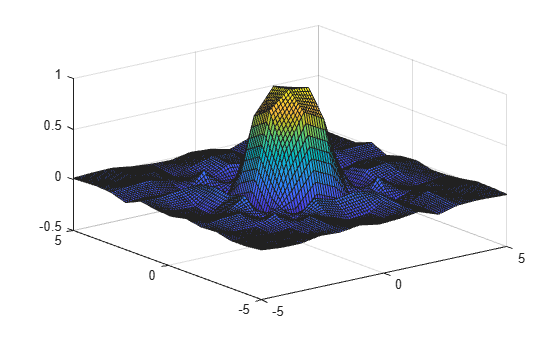In cases where there are a lot of sample points or query points, and where memory usage becomes a concern, you can use grid vectors to improve memory usage.

• When you specify grid vectors instead of using `ndgrid` to create the full grid, `griddedInterpolant` avoids forming the full query grid to carry out the calculations.

• When you pass grid vectors, they are normally grouped together as cells in a cell array, `{xg1, xg2, ..., xgn}`. The grid vectors are a compact way to represent the points of the full grid.Alternatively, execute the previous commands using grid vectors.

```x = -5:0.8:5; y = x'; z = sin(x.^2 + y.^2) ./ (x.^2 + y.^2); F = griddedInterpolant({x,y},z); xq = -5:0.1:5; yq = xq'; vq = F({xq,yq}); surf(xq,yq,vq)```Use the default grid to perform a quick interpolation on a set of sample points. The default grid uses unit-spaced points, so this interpolation is useful when the exact xy spacing between the sample points is not important.

Create a matrix of sample function values and plot them against the default grid.

```x = (1:0.3:5)'; y = x'; V = cos(x) .* sin(y); n = length(x); surf(1:n,1:n,V)```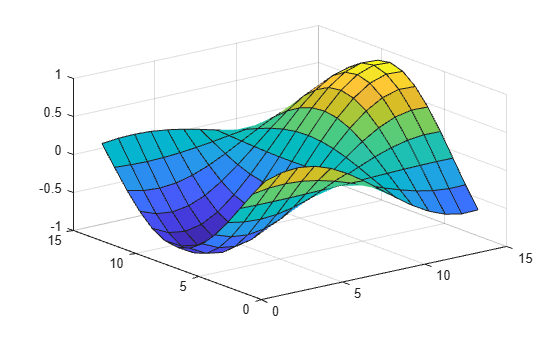Interpolate the data using the default grid.

`F = griddedInterpolant(V)`
```F = griddedInterpolant with properties: GridVectors: {[1 2 3 4 5 6 7 8 9 10 11 12 13 14] [1x14 double]} Values: [14x14 double] Method: 'linear' ExtrapolationMethod: 'linear' ```

Query the interpolant and plot the results.

```[xq,yq] = ndgrid(1:0.2:n); Vq = F(xq,yq); surf(xq',yq',Vq)```Interpolate coarsely sampled data using a full grid with spacing of `0.5`.

Define the sample points as a full grid with range [1, 10] in both dimensions.

`[X,Y] = ndgrid(1:10,1:10);`

Sample $f\left(x,y\right)={x}^{2}+{y}^{2}$ at the grid points.

`V = X.^2 + Y.^2;`

Create the interpolant, specifying cubic interpolation.

`F = griddedInterpolant(X,Y,V,'cubic');`

Define a full grid of query points with `0.5` spacing and evaluate the interpolant at those points. Then plot the result.

```[Xq,Yq] = ndgrid(1:0.5:10,1:0.5:10); Vq = F(Xq,Yq); mesh(Xq,Yq,Vq);```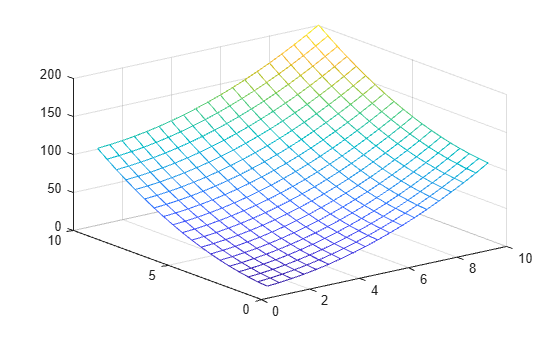Compare results of querying the interpolant outside the domain of `F` using the `'pchip'` and `'nearest'` extrapolation methods.

Create the interpolant, specifying `'pchip'` as the interpolation method and `'nearest'` as the extrapolation method.

```x = [1 2 3 4 5]; v = [12 16 31 10 6]; F = griddedInterpolant(x,v,'pchip','nearest')```
```F = griddedInterpolant with properties: GridVectors: {[1 2 3 4 5]} Values: [12 16 31 10 6] Method: 'pchip' ExtrapolationMethod: 'nearest' ```

Query the interpolant, and include points outside the domain of `F`.

```xq = 0:0.1:6; vq = F(xq); figure plot(x,v,'o',xq,vq,'-b'); legend ('v','vq')```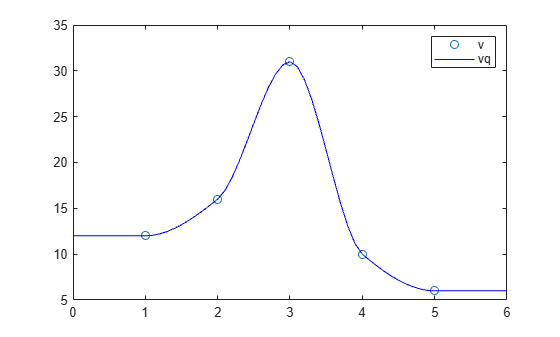Query the interpolant at the same points again, this time using the pchip extrapolation method.

```F.ExtrapolationMethod = 'pchip'; figure vq = F(xq); plot(x,v,'o',xq,vq,'-b'); legend ('v','vq')```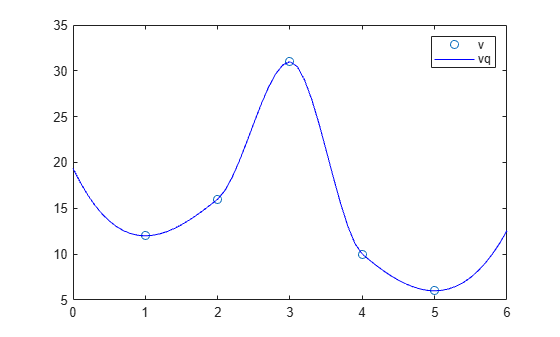expand all

## Tips

• It is quicker to evaluate a `griddedInterpolant` object `F` at many different sets of query points than it is to compute the interpolations separately using `interp1`, `interp2`, `interp3`, or `interpn`. For example:

```% Fast to create interpolant F and evaluate multiple times F = griddedInterpolant(X1,X2,V) v1 = F(Xq1) v2 = F(Xq2) % Slower to compute interpolations separately using interp2 v1 = interp2(X1,X2,V,Xq1) v2 = interp2(X1,X2,V,Xq2) ```